# HeatingResistor

Temperature dependent electrical resistor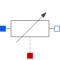# Information

This information is part of the Modelica Standard Library maintained by the Modelica Association.

This is a model for an electrical resistor where the generated heat is dissipated to the environment via connector heatPort and where the resistance R is temperature dependent according to the following equation:

`    R = R_ref*(1 + alpha*(heatPort.T - T_ref))`

alpha is the temperature coefficient of resistance, which is often abbreviated as TCR. In resistor catalogues, it is usually defined as X [ppm/K] (parts per million, similarly to percentage) meaning X*1e-6 [1/K]. Resistors are available for 1 .. 7000 ppm/K, i.e., alpha = 1e-6 .. 7e-3 1/K;

Via parameter useHeatPort the heatPort connector can be enabled and disabled (default = enabled). If it is disabled, the generated heat is transported implicitly to an internal temperature source with a fixed temperature of T_ref.

If the heatPort connector is enabled, it must be connected.

# Parameters (5)

useHeatPort Value: true Type: Boolean Description: =true, if heatPort is enabled Value: T_ref Type: Temperature (K) Description: Fixed device temperature if useHeatPort = false Value: Type: Resistance (Ω) Description: Resistance at temperature T_ref Value: 300.15 Type: Temperature (K) Description: Reference temperature Value: 0 Type: LinearTemperatureCoefficient (¹/K) Description: Temperature coefficient of resistance (R = R_ref*(1 + alpha*(heatPort.T - T_ref))

# Connectors (3)

p n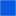Type: PositivePin Description: Positive electrical pin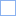Type: NegativePin Description: Negative electrical pin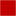Type: HeatPort_a Description: Conditional heat port

# Used in Examples (2)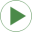HeatingResistor Modelica.Electrical.Analog.Examples Heating resistorControlledTemperature Modelica.Thermal.HeatTransfer.Examples Control temperature of a resistor